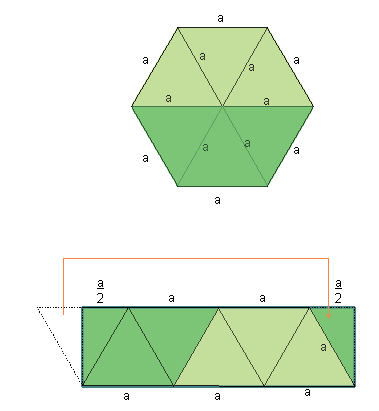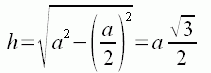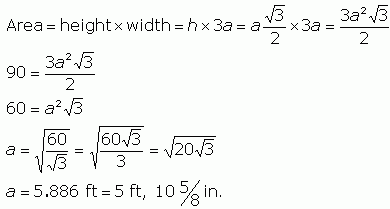SEARCH HOMEMath Central Quandaries & QueriesQuestion from Mark: Trying to buy a tent with 6 sides, in the catalog they give me sq. ft., what is the distance from side to side of a hexagonal tent with an area of 90 sq. ft.Hi Mark.

Let's call the side length a.You can consider the floor of the tent to be equivalent to a rectangular floor when you shift things around, as shown on the right..

If we call the height of the triangle h, then Pythagoras' theorem can express h in terms of the hexagon's side length a:And since you know that the area is 90 sq ft., you can write an expression for the area in terms of a, solve for a and answer your question:Cheers,
Stephen La Rocque.Math Central is supported by the University of Regina and The Pacific Institute for the Mathematical Sciences.